# R S Aggarwal Solutions for Class 11 Maths Chapter 14 Measurement of Angles

R S Aggarwal Solutions given in this section contain detailed step-by-step explanations to all Chapter 14 exercise questions. This chapter in RS Aggarwal Solutions deals with measurement of angles of triangles and the problems related to these angles. Students will also learn about the relationship between degree and radian. This R S Aggarwal study material for Class 11 Maths helps students in mastering all the concepts effectively. Check the detailed R S Aggarwal Solutions for Class 11 chapters and start practicing to score good marks.

## Download PDF of R S Aggarwal Solutions for Class 11 Maths Chapter 14 Measurement of Angles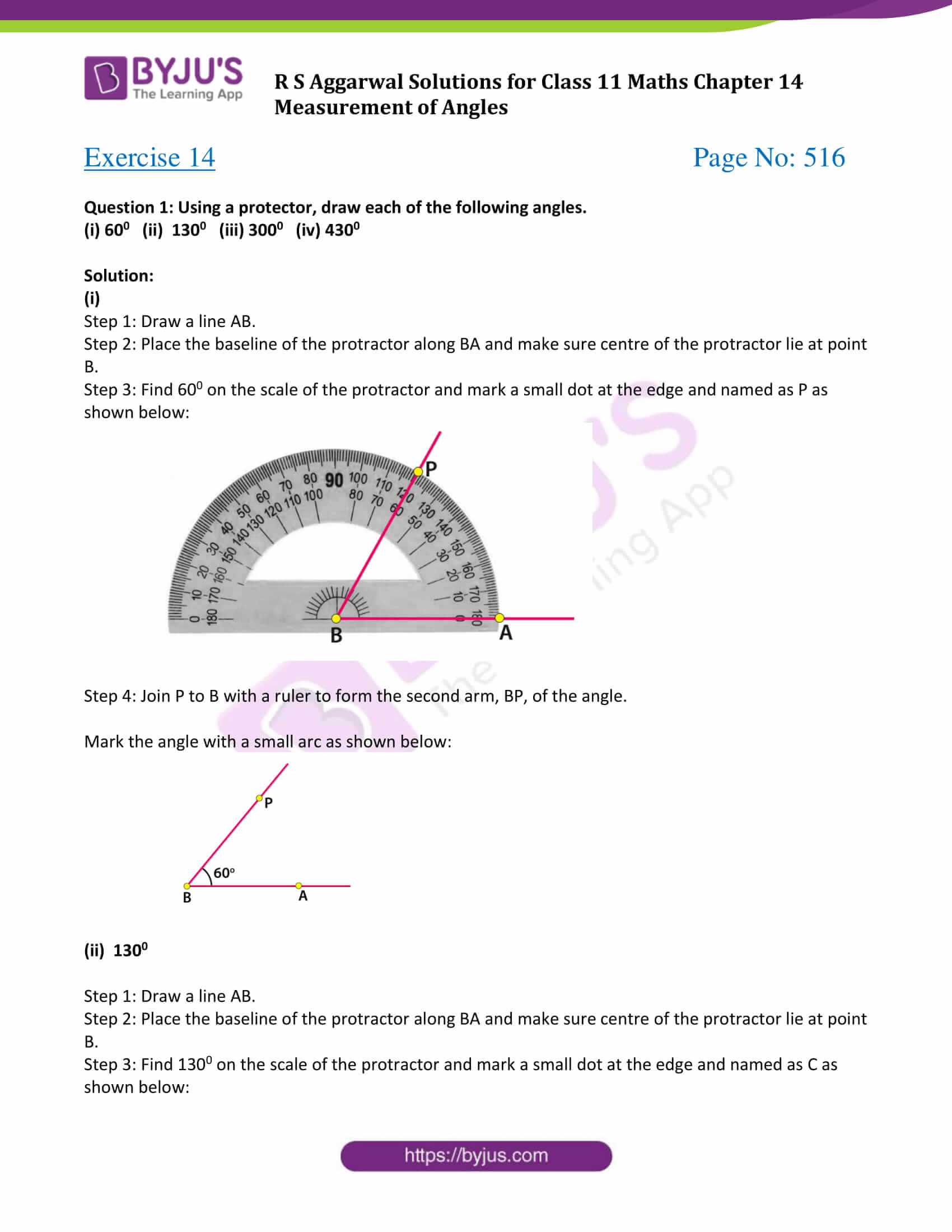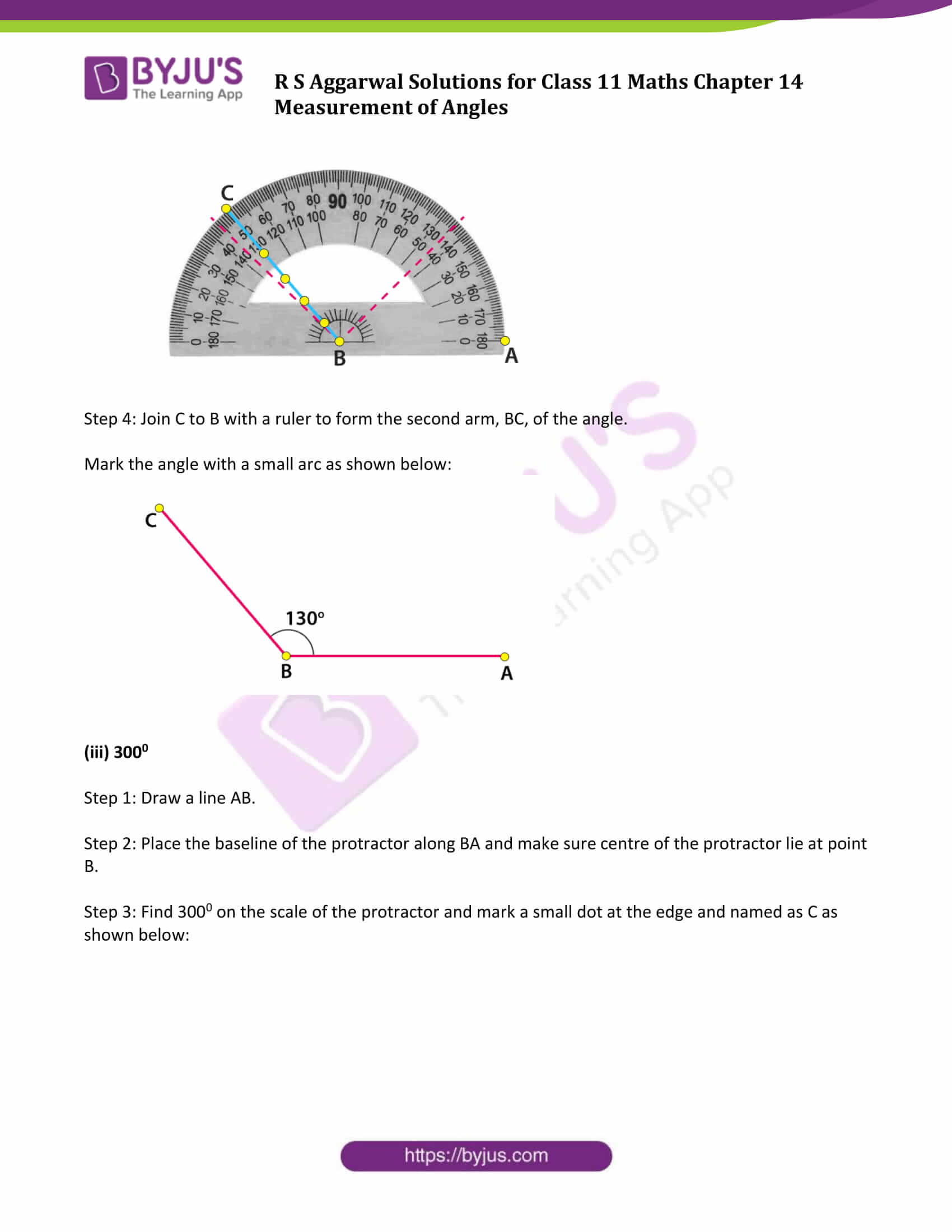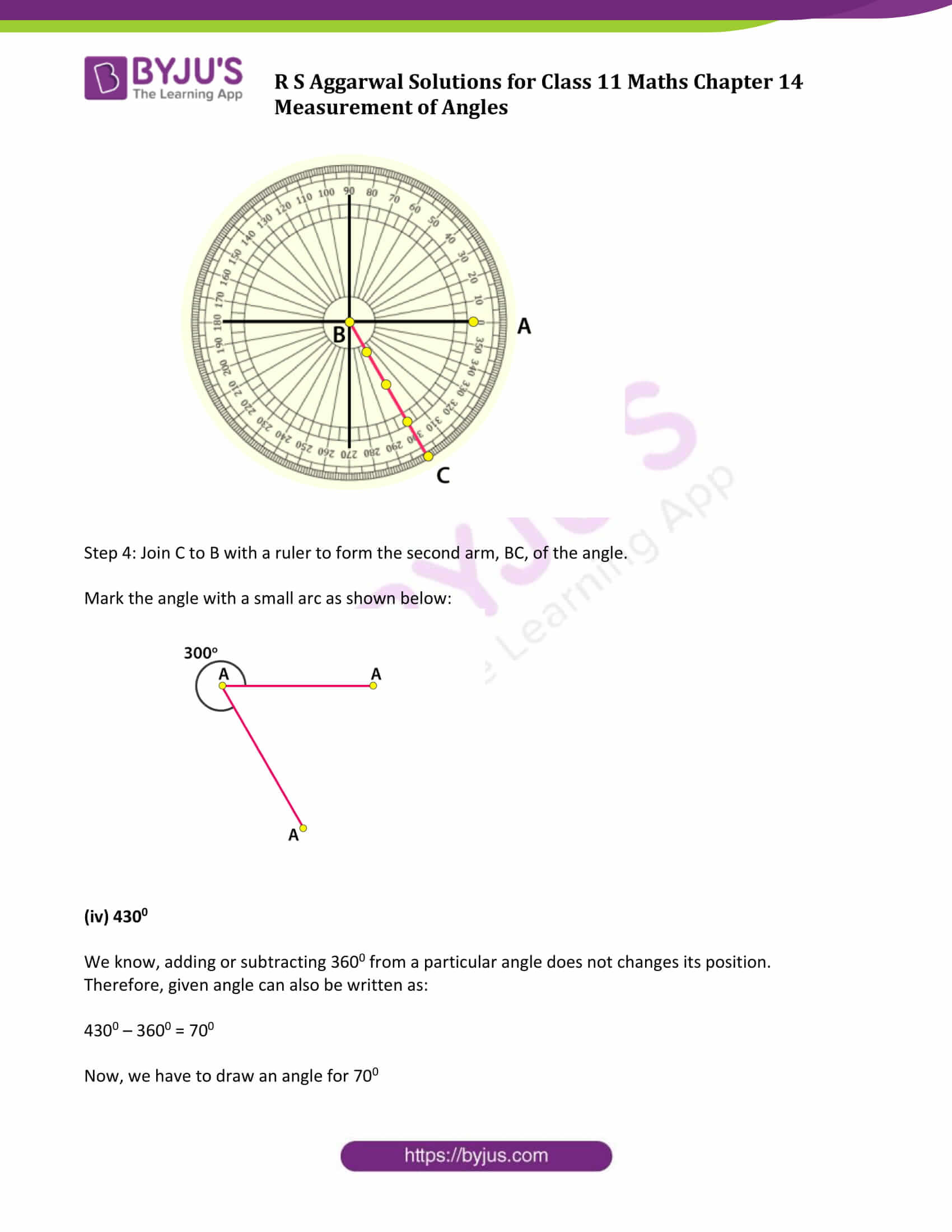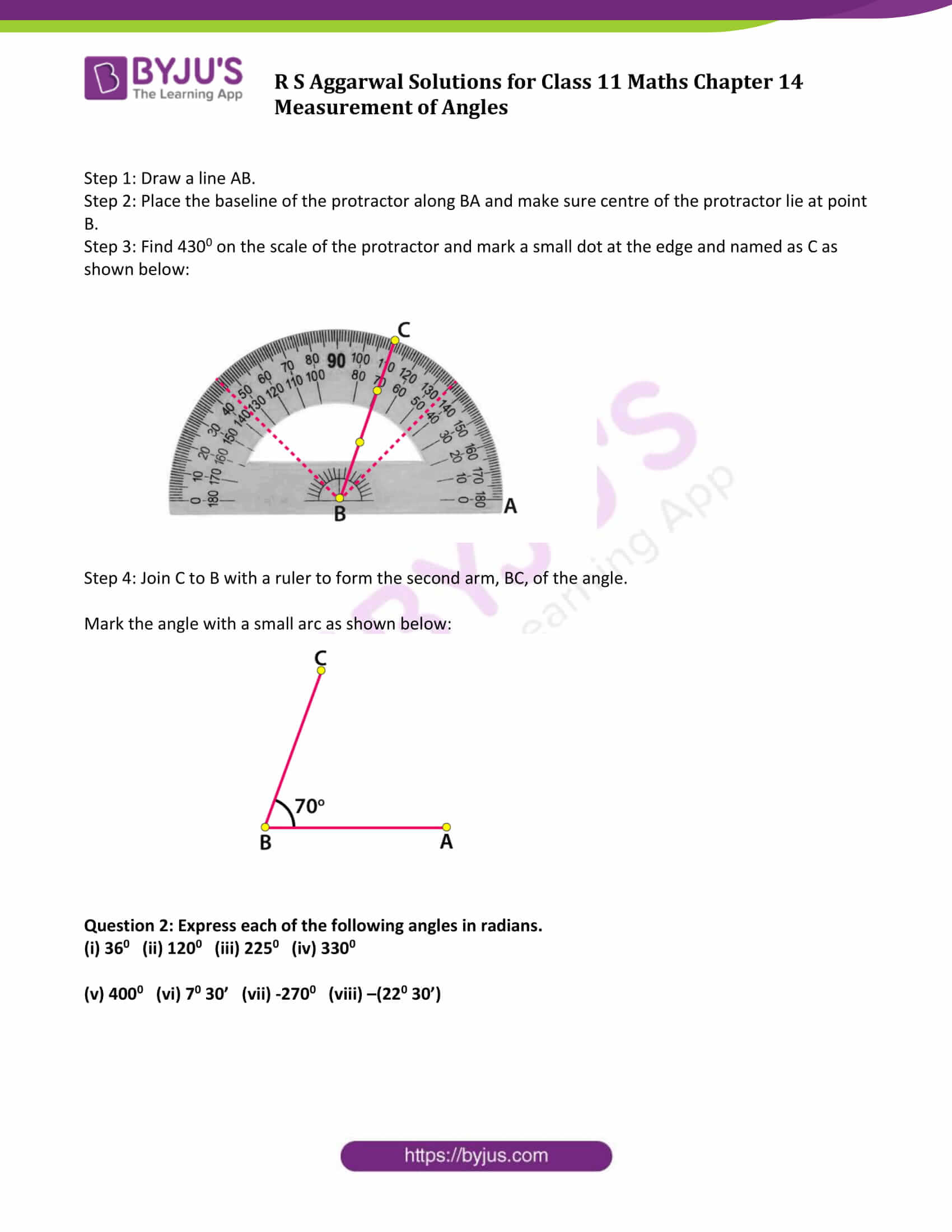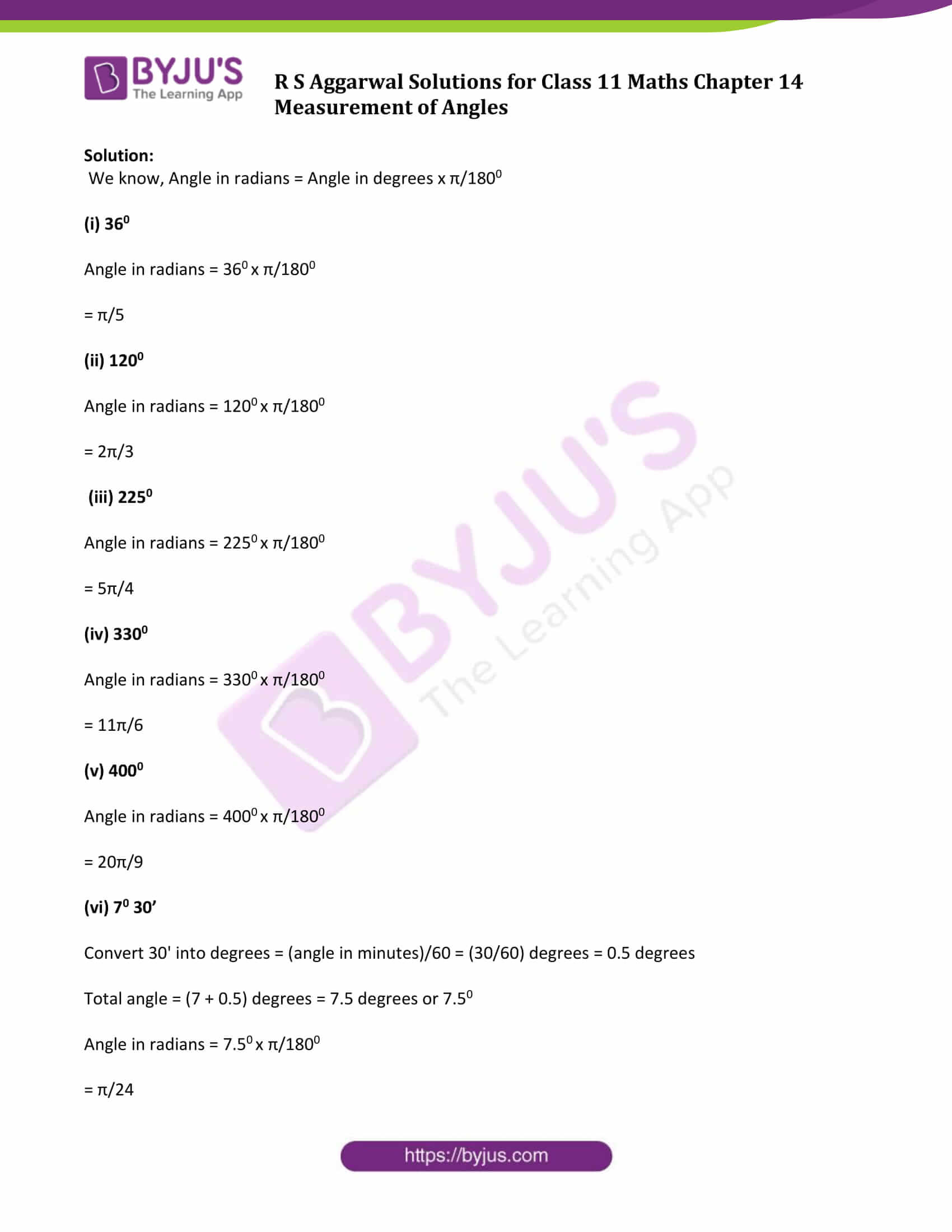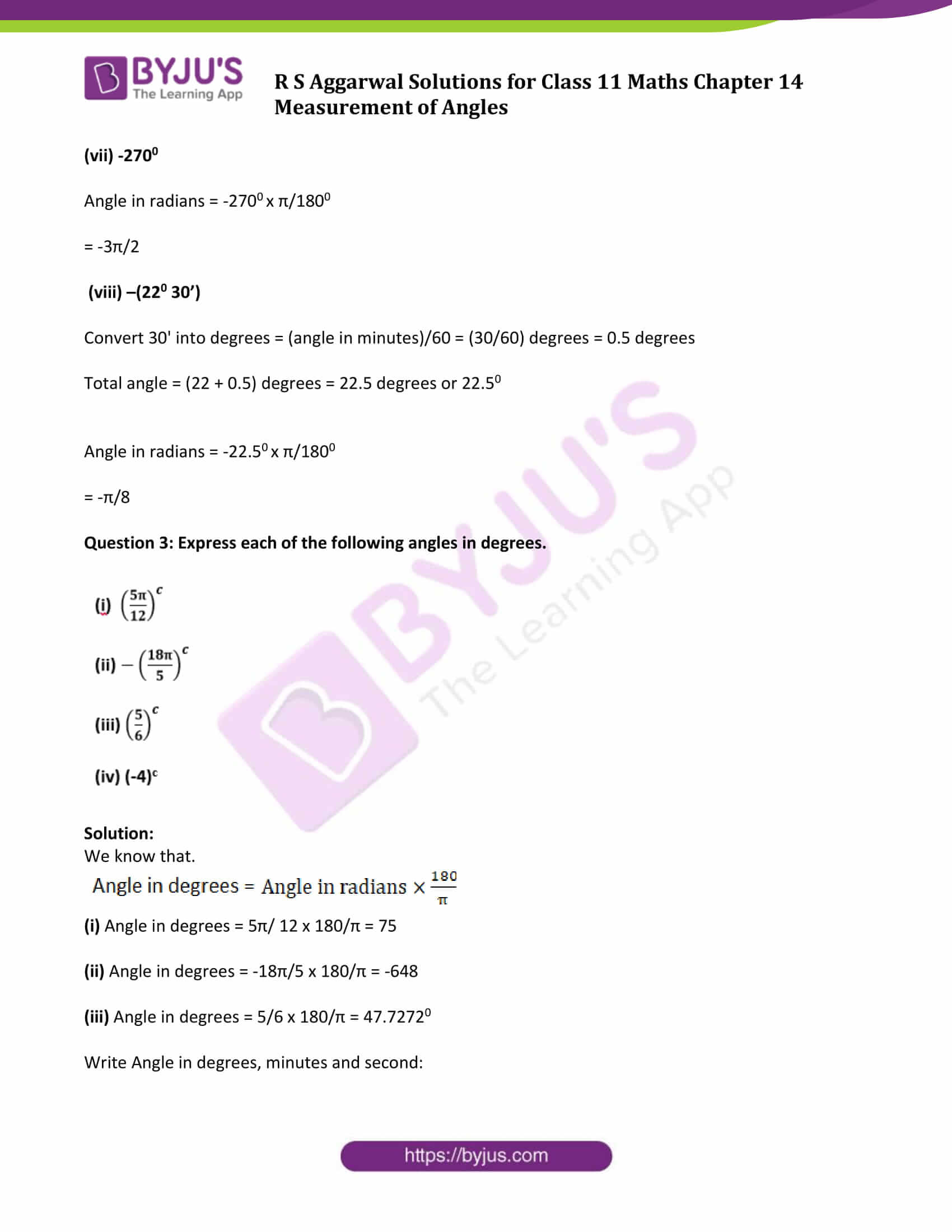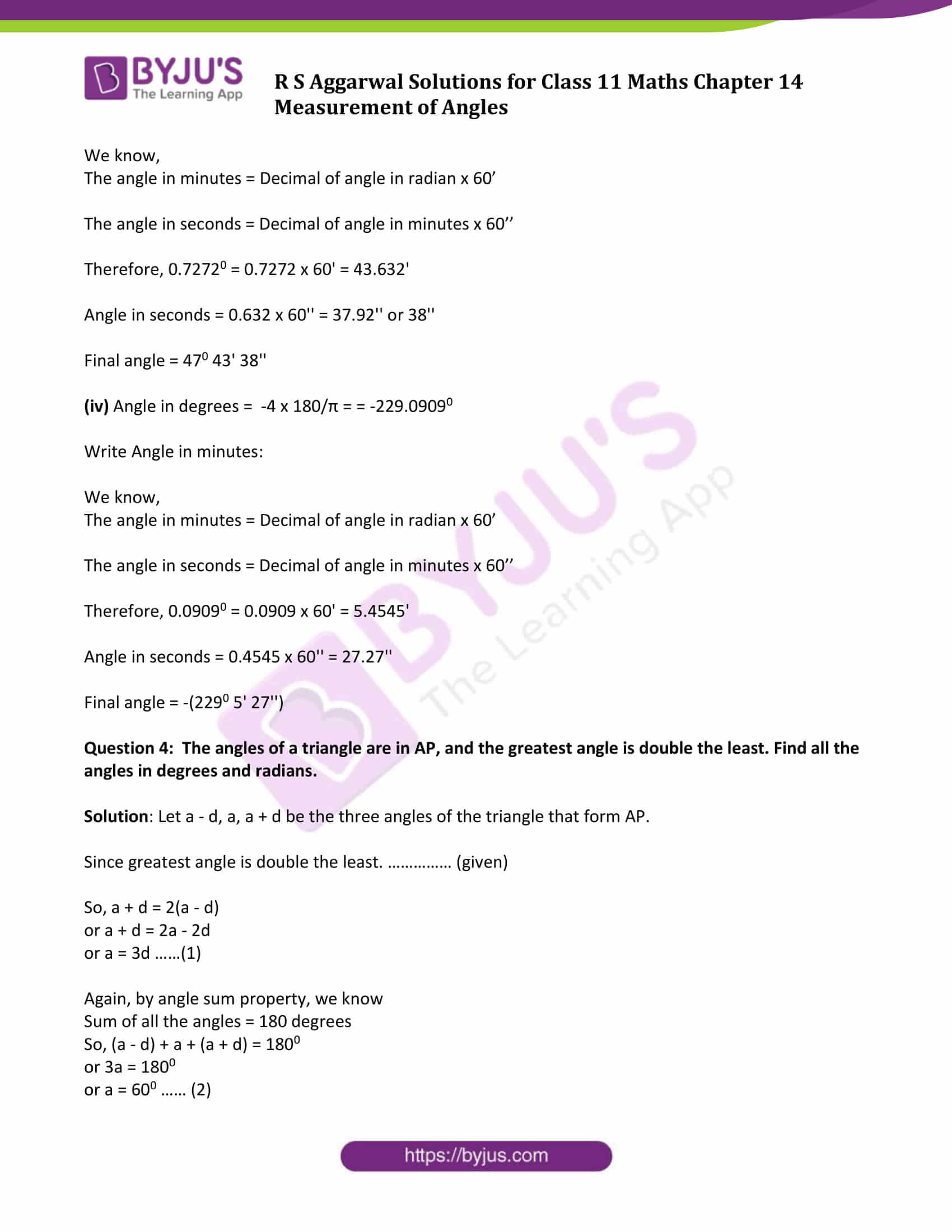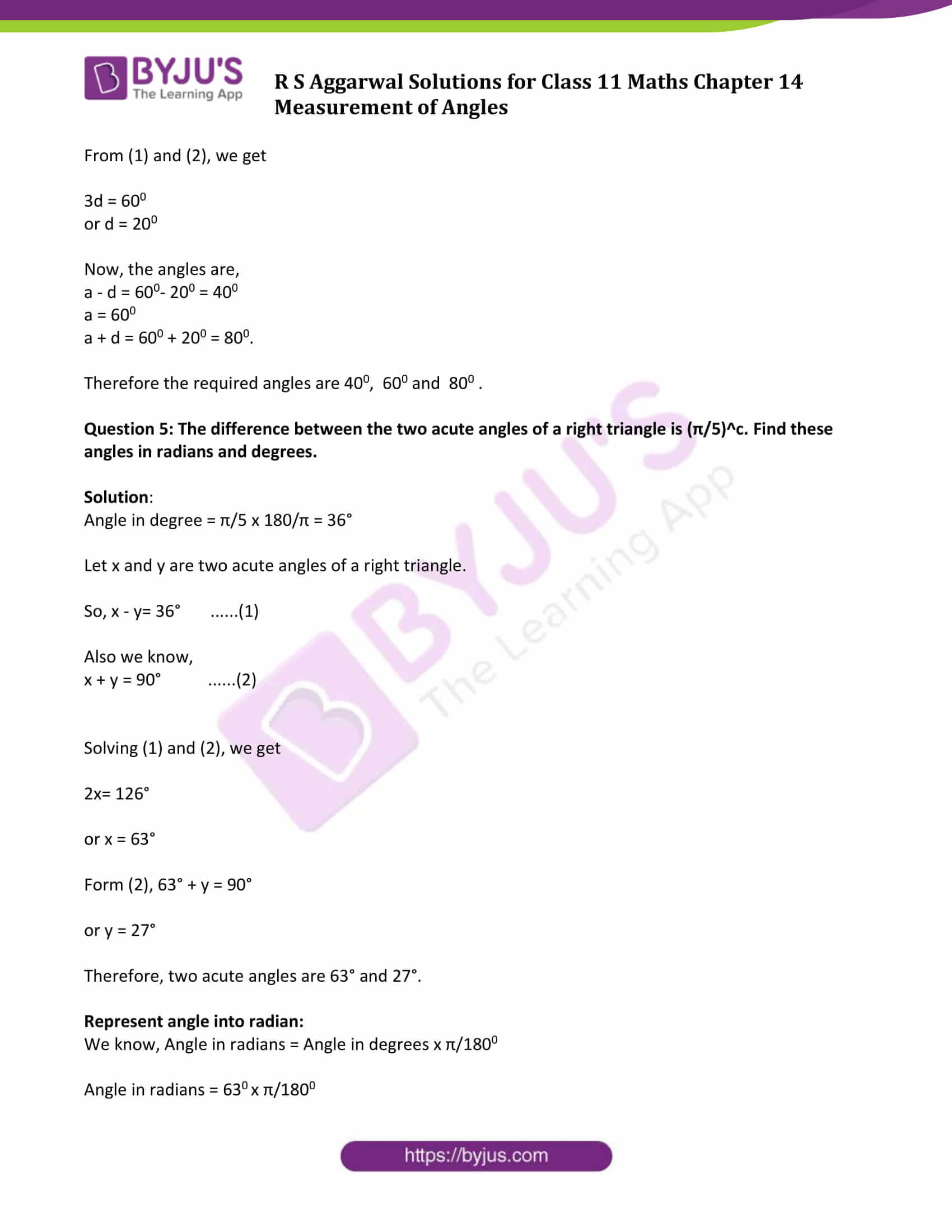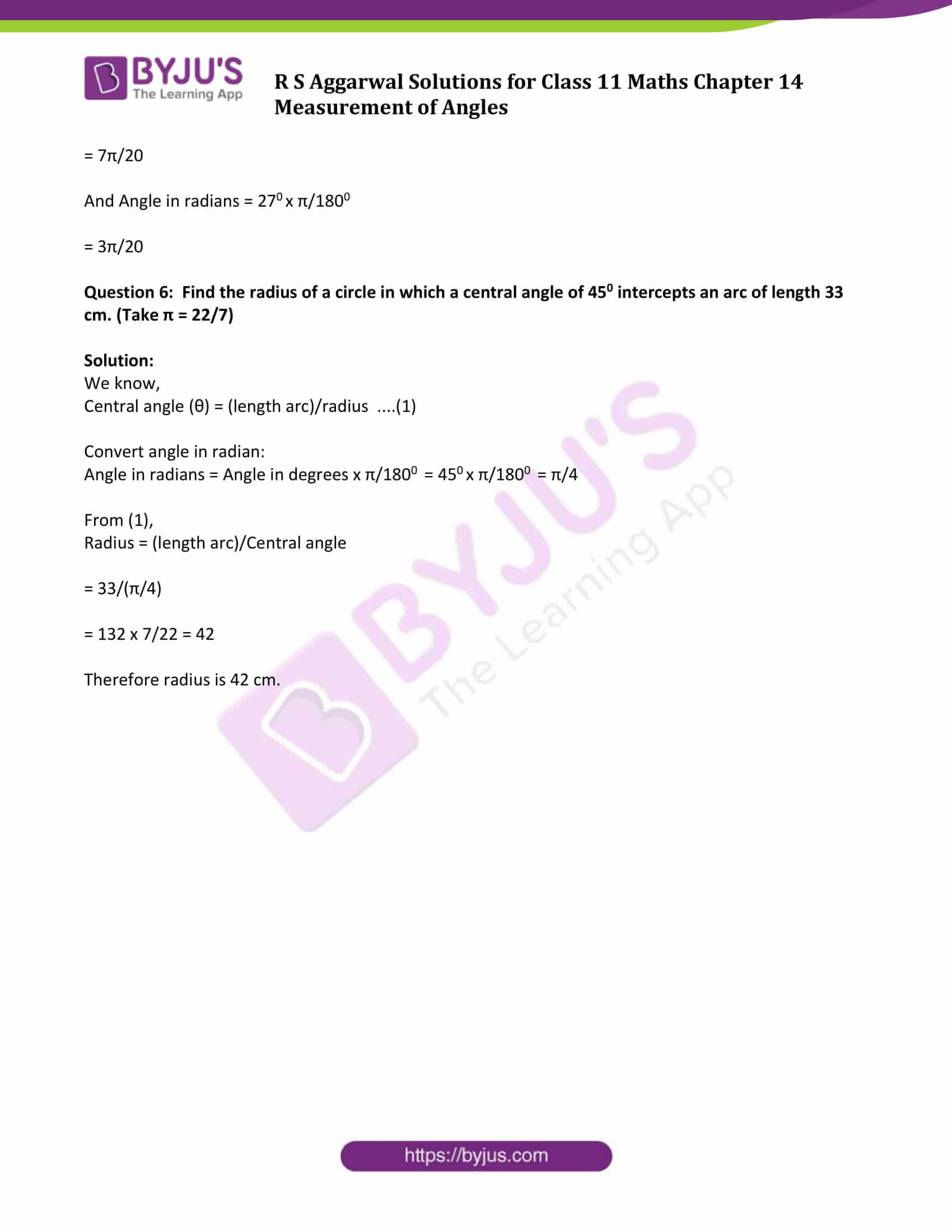### Access Answers to Maths R S Aggarwal Chapter 14 Measurement of Angles

Exercise 14 Page No: 516

Question 1: Using a protector, draw each of the following angles.

(i) 600 (ii) 1300 (iii) 3000 (iv) 4300

Solution:

(i)

Step 1: Draw a line AB.

Step 2: Place the baseline of the protractor along BA and make sure centre of the protractor lie at point B.

Step 3: Find 600 on the scale of the protractor and mark a small dot at the edge and named as P as shown below: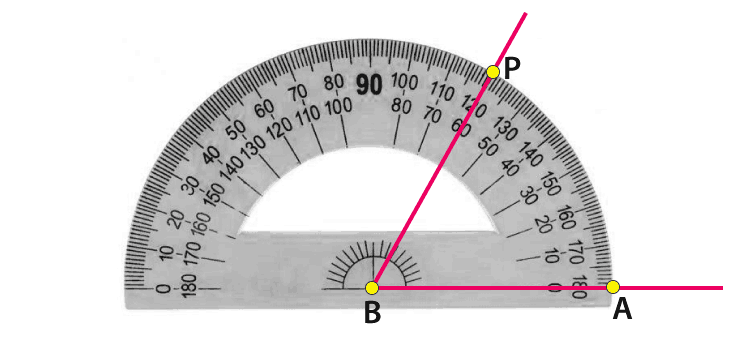Step 4: Join P to B with a ruler to form the second arm, BP, of the angle.

Mark the angle with a small arc as shown below: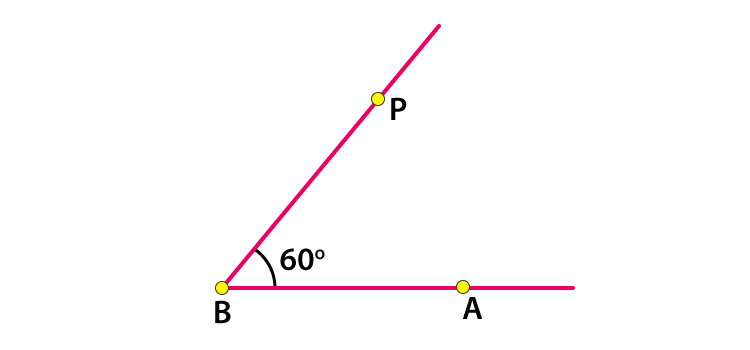(ii) 1300

Step 1: Draw a line AB.

Step 2: Place the baseline of the protractor along BA and make sure centre of the protractor lie at point B.

Step 3: Find 1300 on the scale of the protractor and mark a small dot at the edge and named as C as shown below: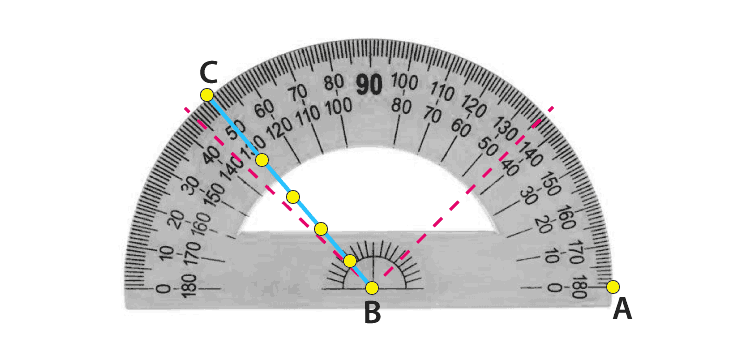Step 4: Join C to B with a ruler to form the second arm, BC, of the angle.

Mark the angle with a small arc as shown below: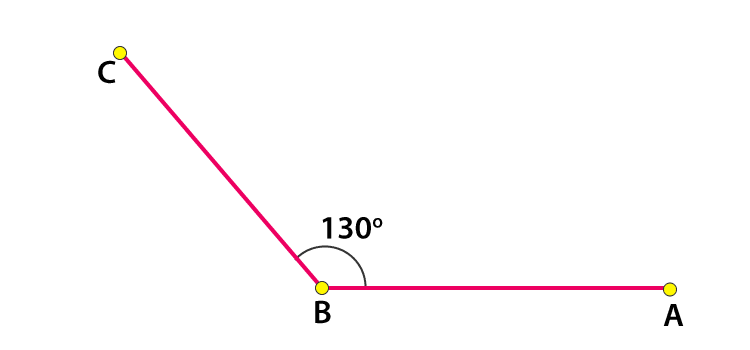(iii) 3000

Step 1: Draw a line AB.

Step 2: Place the baseline of the protractor along BA and make sure centre of the protractor lie at point B.

Step 3: Find 3000 on the scale of the protractor and mark a small dot at the edge and named as C as shown below: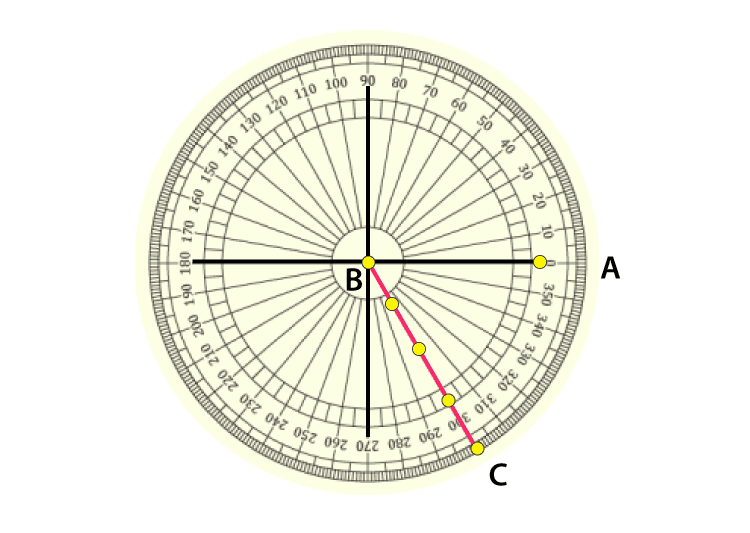Step 4: Join C to B with a ruler to form the second arm, BC, of the angle.

Mark the angle with a small arc as shown below: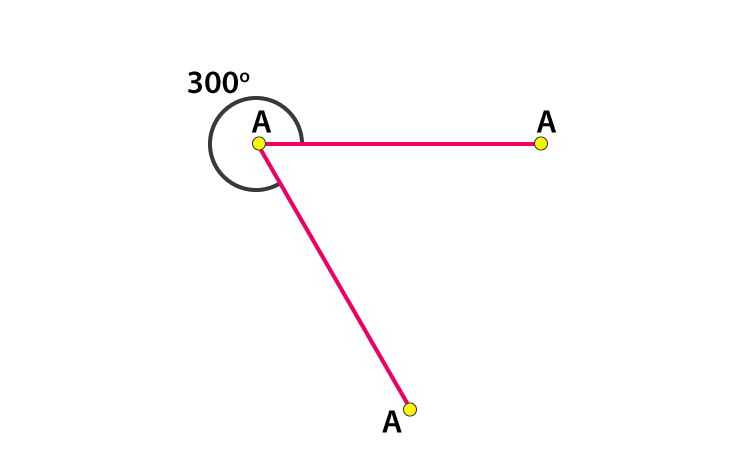(iv) 4300

We know, adding or subtracting 3600 from a particular angle does not changes its position.

Therefore, given angle can also be written as:

4300 – 3600 = 700

Now, we have to draw an angle for 700

Step 1: Draw a line AB.

Step 2: Place the baseline of the protractor along BA and make sure centre of the protractor lie at point B.

Step 3: Find 4300 on the scale of the protractor and mark a small dot at the edge and named as C as shown below: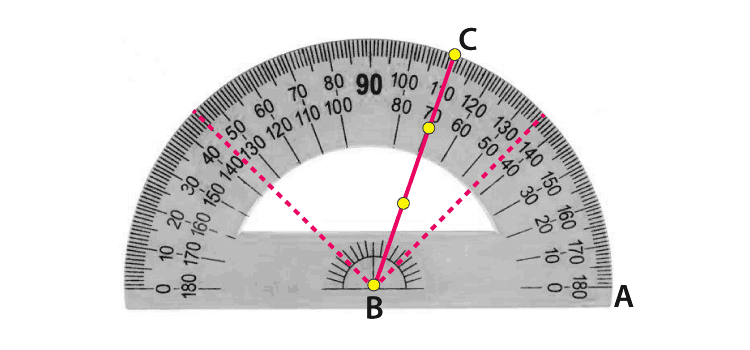Step 4: Join C to B with a ruler to form the second arm, BC, of the angle.

Mark the angle with a small arc as shown below: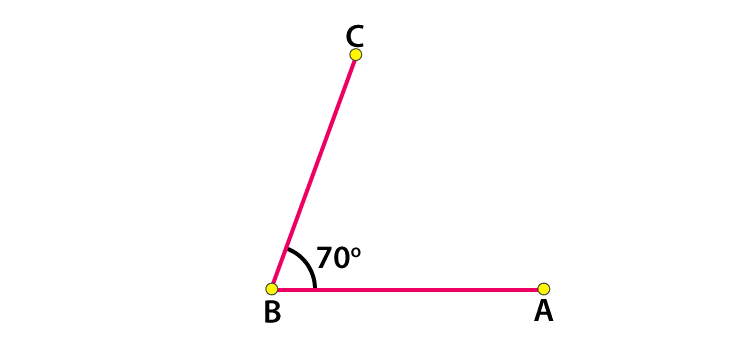Question 2: Express each of the following angles in radians.

(i) 360 (ii) 1200 (iii) 2250 (iv) 3300

(v) 4000 (vi) 70 30’ (vii) -2700 (viii) –(220 30’)

Solution:

We know, Angle in radians = Angle in degrees x π/1800

(i) 360

Angle in radians = 360 x π/1800

= π/5

(ii) 1200

Angle in radians = 1200 x π/1800

= 2π/3

(iii) 2250

Angle in radians = 2250 x π/1800

= 5π/4

(iv) 3300

Angle in radians = 3300 x π/1800

= 11π/6

(v) 4000

Angle in radians = 4000 x π/1800

= 20π/9

(vi) 70 30’

Convert 30′ into degrees = (angle in minutes)/60 = (30/60) degrees = 0.5 degrees

Total angle = (7 + 0.5) degrees = 7.5 degrees or 7.50

Angle in radians = 7.50 x π/1800

= π/24

(vii) -2700

Angle in radians = -2700 x π/1800

= -3π/2

(viii) –(220 30’)

Convert 30′ into degrees = (angle in minutes)/60 = (30/60) degrees = 0.5 degrees

Total angle = (22 + 0.5) degrees = 22.5 degrees or 22.50

Angle in radians = -22.50 x π/1800

= -π/8

Question 3: Express each of the following angles in degrees.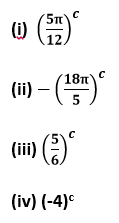Solution:

We know that.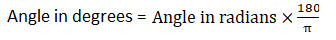(i) Angle in degrees = 5π/ 12 x 180/π = 75

(ii) Angle in degrees = -18π/5 x 180/π = -648

(iii) Angle in degrees = 5/6 x 180/π = 47.72720

Write Angle in degrees, minutes and second:

We know,

The angle in minutes = Decimal of angle in radian x 60’

The angle in seconds = Decimal of angle in minutes x 60’’

Therefore, 0.72720 = 0.7272 x 60′ = 43.632′

Angle in seconds = 0.632 x 60” = 37.92” or 38”

Final angle = 470 43′ 38”

(iv) Angle in degrees = -4 x 180/π = = -229.09090

Write Angle in minutes:

We know,

The angle in minutes = Decimal of angle in radian x 60’

The angle in seconds = Decimal of angle in minutes x 60’’

Therefore, 0.09090 = 0.0909 x 60′ = 5.4545′

Angle in seconds = 0.4545 x 60” = 27.27”

Final angle = -(2290 5′ 27”)

Question 4: The angles of a triangle are in AP, and the greatest angle is double the least. Find all the angles in degrees and radians.

Solution: Let a – d, a, a + d be the three angles of the triangle that form AP.

Since greatest angle is double the least. …………… (given)

So, a + d = 2(a – d)

or a + d = 2a – 2d

or a = 3d ……(1)

Again, by angle sum property, we know

Sum of all the angles = 180 degrees

So, (a – d) + a + (a + d) = 1800

or 3a = 1800

or a = 600 …… (2)

From (1) and (2), we get

3d = 600

or d = 200

Now, the angles are,

a – d = 600– 200 = 400

a = 600

a + d = 600 + 200 = 800.

Therefore the required angles are 400, 600 and 800 .

Question 5: The difference between the two acute angles of a right triangle is (π/5)^c. Find these angles in radians and degrees.

Solution:

Angle in degree = π/5 x 180/π = 36°

Let x and y are two acute angles of a right triangle.

So, x – y= 36° ……(1)

Also we know,

x + y = 90° ……(2)

Solving (1) and (2), we get

2x= 126°

or x = 63°

Form (2), 63° + y = 90°

or y = 27°

Therefore, two acute angles are 63° and 27°.

We know, Angle in radians = Angle in degrees x π/1800

Angle in radians = 630 x π/1800

= 7π/20

And Angle in radians = 270 x π/1800

= 3π/20

Question 6: Find the radius of a circle in which a central angle of 450 intercepts an arc of length 33 cm. (Take π = 22/7)

Solution:

We know,

Central angle (θ) = (length arc)/radius ….(1)

Angle in radians = Angle in degrees x π/1800 = 450 x π/1800 = π/4

From (1),

= 33/(π/4)

= 132 x 7/22 = 42